In the given figure each plate of capacitance C has partial value of charge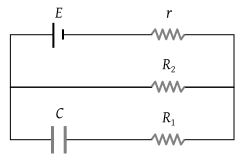(1) CE

(2) $\frac{CE{R}_{1}}{{R}_{2}-r}$

(3) $\frac{CE{R}_{2}}{{R}_{2}+r}$

(4) $\frac{CE{R}_{1}}{{R}_{1}-r}$

Concept Questions :-

RC circuit
High Yielding Test Series + Question Bank - NEET 2020

Difficulty Level:

The plates of a capacitor are charged to a potential difference of 320 volts and are then connected across a resistor. The potential difference across the capacitor decays exponentially with time. After 1 second the potential difference between the plates of the capacitor is 240 volts, then after 2 and 3 seconds the potential difference between the plates will be

(1) 200 and 180 V

(2) 180 and 135 V

(3) 160 and 80 V

(4) 140 and 20 V

Concept Questions :-

RC circuit
High Yielding Test Series + Question Bank - NEET 2020

Difficulty Level:

The plates of a parallel plate condenser are pulled apart with a velocity v. If at any instant their mutual distance of separation is d, then the magnitude of the time of rate of change of capacity depends on d as follows

(1) 1/d

(2) 1/d2

(3) d2

(4) d

Concept Questions :-

Types of capactiors
High Yielding Test Series + Question Bank - NEET 2020

Difficulty Level:

A fully charged capacitor has a capacitance ‘C’. It is discharged through a small coil of resistance wire embedded in a thermally insulated block of specific heat capacity ‘s’ and mass ‘m’. If the temperature of the block is raised by ‘ΔT’, the potential difference ‘V’ across the capacitance is

(1) $\frac{ms\Delta T}{C}$

(2) $\sqrt{\frac{2ms\Delta T}{C}}$

(3) $\sqrt{\frac{2mC\Delta T}{s}}$

(4) $\frac{mC\Delta T}{s}$

Concept Questions :-

Energy stored in capacitor
High Yielding Test Series + Question Bank - NEET 2020

Difficulty Level:

A network of four capacitors of capacity equal to ${C}_{1}=C,\text{\hspace{0.17em}\hspace{0.17em}}{C}_{2}=2C,\text{\hspace{0.17em}}{C}_{3}=3C$ and C4 = 4 C are connected in a battery as shown in the figure. The ratio of the charges on C2 and C4 is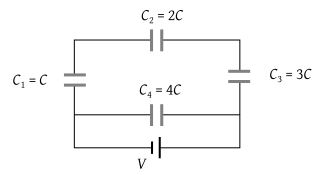(1) $\frac{22}{3}$

(2) $\frac{3}{22}$

(3) $\frac{7}{4}$

(4) $\frac{4}{7}$

Concept Questions :-

Combination of capactiors
High Yielding Test Series + Question Bank - NEET 2020

Difficulty Level:

A 4 μF capacitor, a resistance of 2.5 MΩ is in series with 12 V battery. Find the time after which the potential difference across the capacitor is 3 times the potential difference across the resistor. [Given ln(2)= 0.693]

(1) 13.86 s

(2) 6.93 s

(3) 7 s

(4) 14 s

Concept Questions :-

RC circuit
High Yielding Test Series + Question Bank - NEET 2020

Difficulty Level:

The variation of potential with distance R from a fixed point is as shown below. The electric field at R = 5 m is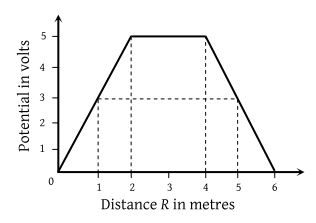(1) 2.5 volt/m

(2) –2.5 volt/m

(3) 2/5 volt/m

(4) –2/5 volt/m

Concept Questions :-

Relation between field and potential
High Yielding Test Series + Question Bank - NEET 2020

Difficulty Level:

The figure gives the electric potential V as a function of distance through five regions on x-axis. Which of the following is true for the electric field E in these regions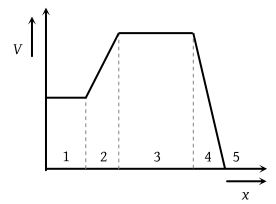(1) ${E}_{1}>{E}_{2}>{E}_{3}>{E}_{4}>{E}_{5}$

(2) ${E}_{1}={E}_{3}={E}_{5}$ and ${E}_{2}<{E}_{4}$

(3) ${E}_{2}={E}_{4}={E}_{5}$ and ${E}_{1}<{E}_{3}$

(4) ${E}_{1}<{E}_{2}<{E}_{3}<{E}_{4}<{E}_{5}$

Concept Questions :-

Relation between field and potential
High Yielding Test Series + Question Bank - NEET 2020

Difficulty Level:

In a hollow spherical shell potential (V) changes with respect to distance (r) from centre

(1)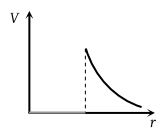(2)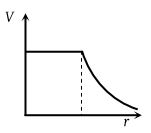(3)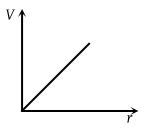(4)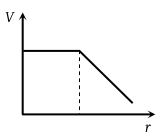Concept Questions :-

Electric potential
High Yielding Test Series + Question Bank - NEET 2020

Difficulty Level:

What physical quantities may X and Y represent ? (Y represents the first mentioned quantity)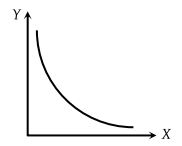(1) Pressure v/s temperature of a given gas (constant volume)

(2) Kinetic energy v/s velocity of a particle

(3) Capacitance v/s charge to give a constant potential

(4) Potential v/s capacitance to give a constant charge

Concept Questions :-

Capacitance